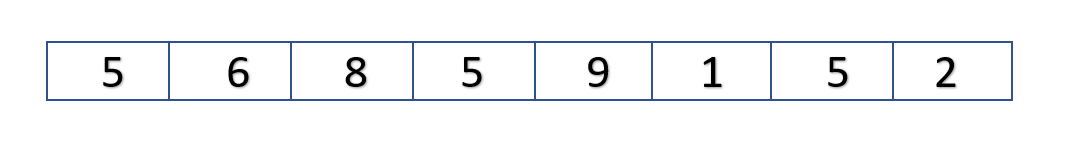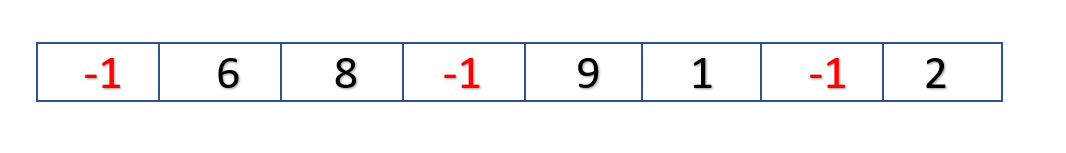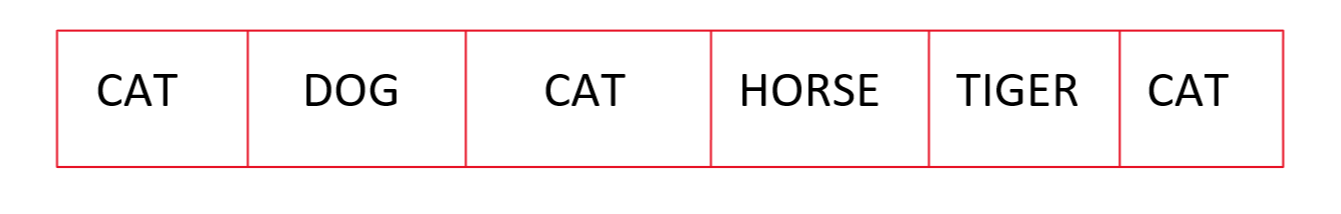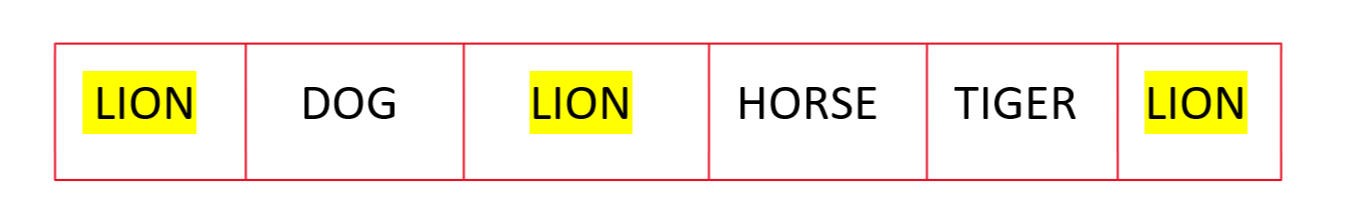Open in App
Not now

# Replacing All Occurrences of Specified Element of Java Vector

• Last Updated : 28 Dec, 2020

The replaceAll() method of java.util.Collections class is used to Replaces all occurrences of one specified value in a list with another. More formally, replaces with newVal each element e in list such that (oldVal==null ? e==null : oldVal.equals(e)). (This method has no effect on the size of the list.)

Let’s consider the following vector:VECTOR BEFORE REPLACING

Now in this vector we have to replace all occurrences of 5 with a given value. Let’s say the value here is -1. After replacing our vector should become as shown below:VECTOR AFTER REPLACING

Approach:

A naive way to approach this problem is to traverse the entire vector and while traversal if the element in vector is equal the specified element then replace it with the given value.

However, in Java, we have a Built-in method  replaceAll() as a part of Java Collections which does the same.

Syntax:

```public static  boolean
replaceAll(List list, T oldVal, T newVal)```

Parameters: This method takes the following argument as a Parameter

• list – the list in which replacement is to occur.
• oldVal – the old value to be replaced.
• newVal – the new value with which oldVal is to be replaced.

Return Value: This method returns true if list contained one or more elements e such that (oldVal==null ? e==null : oldVal.equals(e)).

Code:

## Java

 `// Java program to replace all occurrences``// of Specified Element of Java Vector`` ` `import` `java.io.*;``import` `java.util.Vector;``import` `java.util.Collections;``class` `GFG {``    ``public` `static` `void` `main (String[] args) ``    ``{``      ``// Create a vector``      ``Vector storage =``new` `Vector(``6``);``       ` `      ``// adding elements to the vector``      ``storage.add(``5``);      ``      ``storage.add(``6``);``      ``storage.add(``8``);``      ``storage.add(``5``);``      ``storage.add(``9``);``      ``storage.add(``1``);      ``      ``storage.add(``5``);``      ``storage.add(``2``);`` ` `       ` `      ``// val to replace with ``      ``int` `val=-``1``;``       ` `       ` `      ``// printing the vector before replacing ``      ``System.out.println(``"Vector before Replacing is: "` `+ storage);``       ` `      ``// using Collections.replaceAll to replace all occurrences of the element``      ``Collections.replaceAll(storage,``5``,val);``       ` `       ` `      ``//printing the vector after replacing ``      ``System.out.println(``"Vector after Replacing is:  "` `+ storage);``      ` `       ` `       ` `    ``}``}`

Output

```Vector before Replacing is: [5, 6, 8, 5, 9, 1, 5, 2]
Vector after Replacing is:  [-1, 6, 8, -1, 9, 1, -1, 2]```

Now let’s consider a Vector having String instead of Integer values:VECTOR BEFORE REPLACING

Now in this vector, we have to replace all occurrences of CAT with a given String. Let’s say the string here is LION. After replacing our vector should become as shown below:VECTOR AFTER REPLACING

Code:

## Java

 `// Java program to replace all occurrences``// of Specified Element of Java Vector`` ` `import` `java.io.*;``import` `java.util.Vector;``import` `java.util.Collections;``class` `GFG {``    ``public` `static` `void` `main (String[] args) {``      ``// Create a vector``      ``Vector storage =``new` `Vector(``6``);``      ``// adding elements to the vector``      ``storage.add(``"CAT"``);      ``      ``storage.add(``"DOG"``);``      ``storage.add(``"CAT"``);``      ``storage.add(``"HORSE"``);``      ``storage.add(``"TIGER"``);``      ``storage.add(``"CAT"``);``       ` `      ``// val to replace with ``     ``String val=``"LION"``;``       ` `       ` `      ``// printing the vector before replacing ``      ``System.out.println(``"Vector before Replacing is: "` `+ storage);``       ` `      ``// using Collections.replaceAll to replace all occurrences of specified  element``      ``Collections.replaceAll(storage,``"CAT"``,val);``       ` `       ` `      ``//printing the vector after replacing ``      ``System.out.println(``"Vector after Replacing is:  "` `+ storage);``      ` `       ` `       ` `    ``}``}`

Output

```Vector before Replacing is: [CAT, DOG, CAT, HORSE, TIGER, CAT]
Vector after Replacing is:  [LION, DOG, LION, HORSE, TIGER, LION]```

My Personal Notes arrow_drop_up